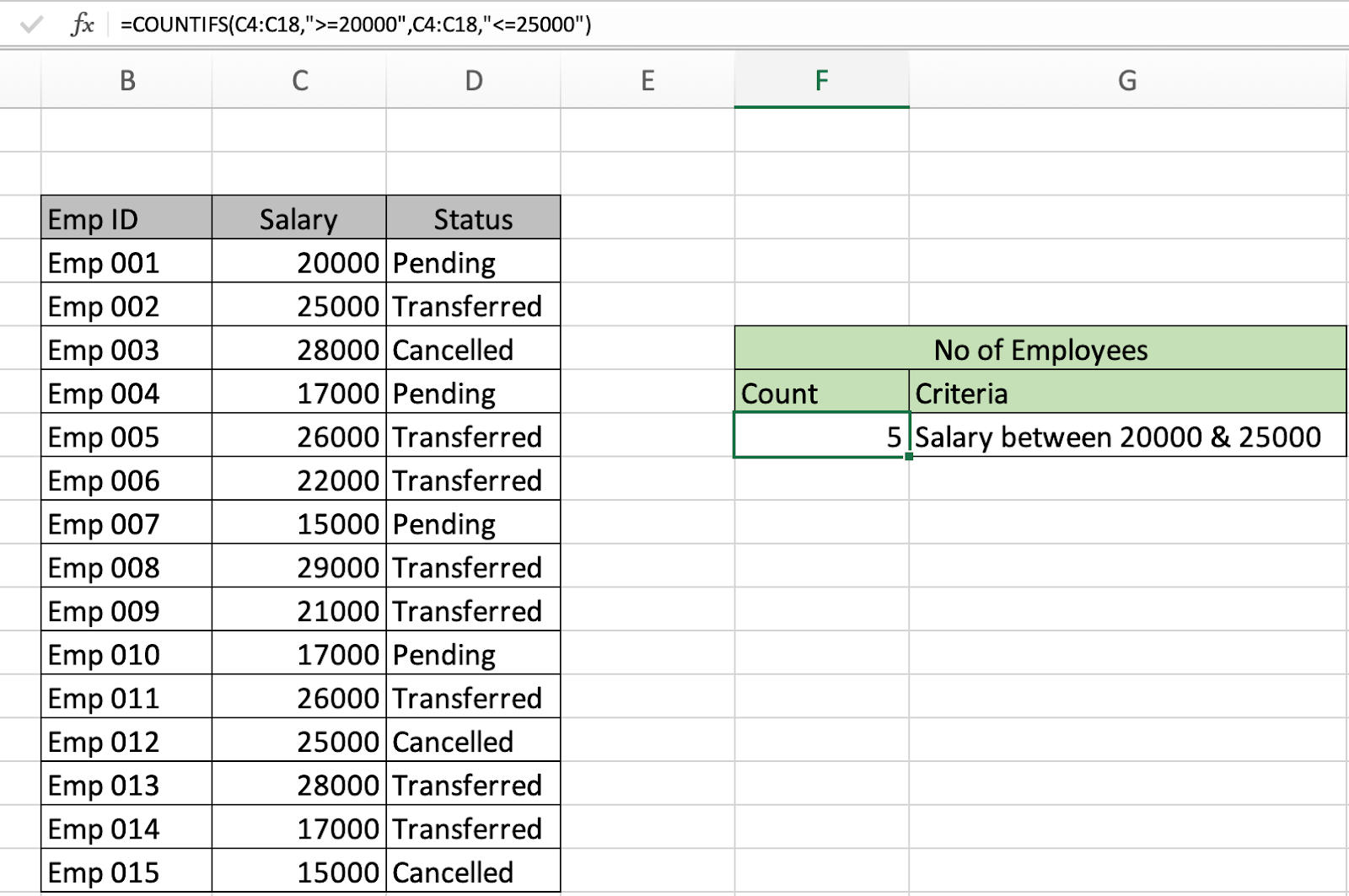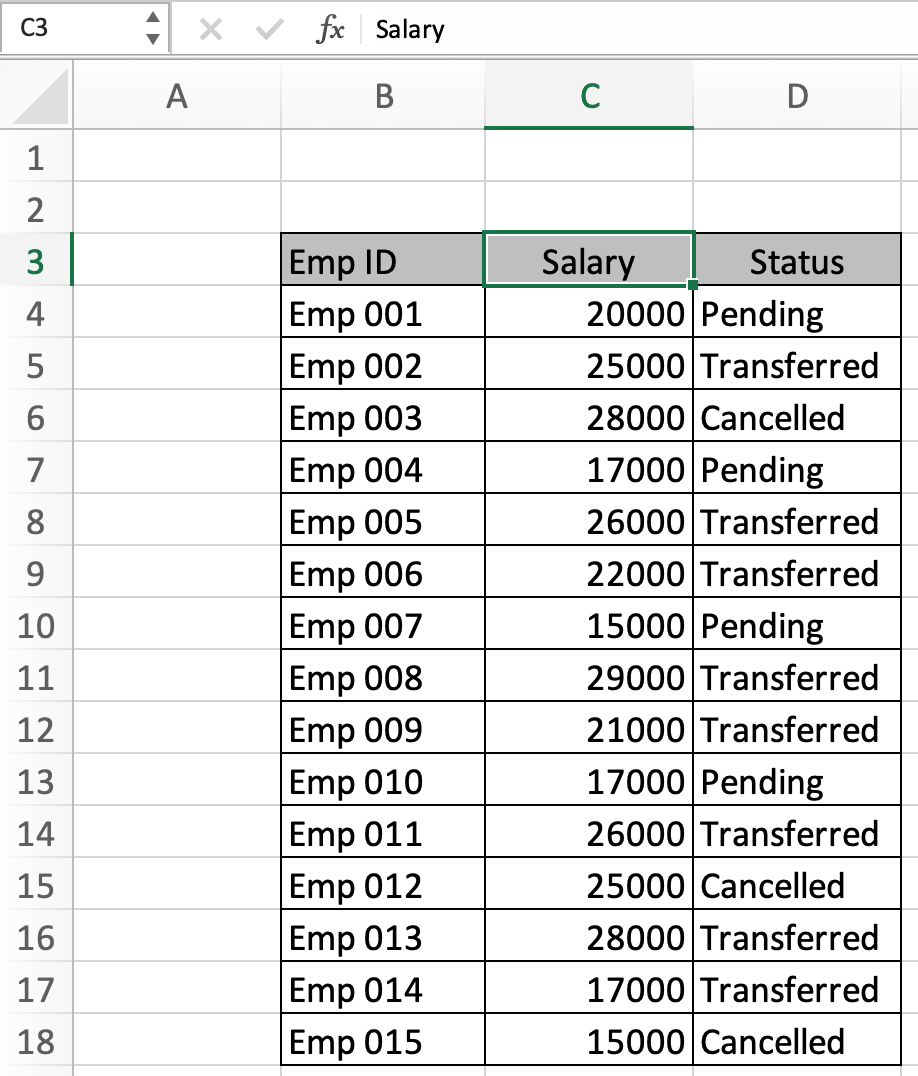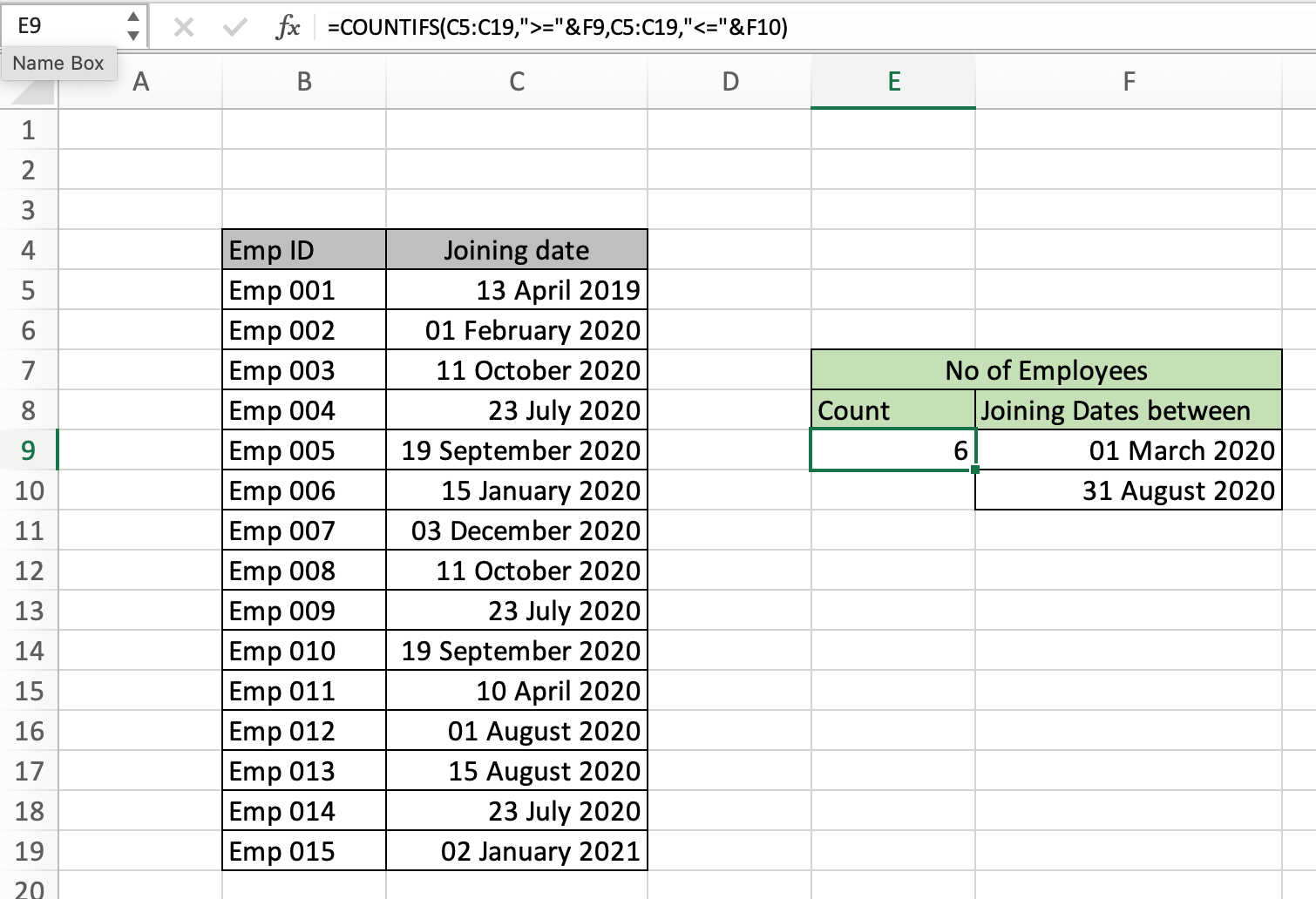# How to count cells between values in ExcelIn this article, we will learn How to count cells between values in Excel.

Count number of records matching multiple criteria

In Excel, whenever we require to count cells which satisfy more than one criteria we use the COUNTIFS function in Excel. For example countif between two numbers or two dates or any numerical list. You can either count it manually if the list length is small, but it's not possible for long lists. Let's understand how to use the COUNTIFS function to count records between given any two values.

COUNTIFS formula in Excel

 =COUNTIFS(criteria_range1, criteria1, criteria_range2, criteria2)

criteria_range1: This is the first criteria list or range.

criteria1: This is the first criteria on criteria range1.

criteria_range2: This is the second criteria list or range.

criteria2: The criteria2 is the second condition from criteria range 2.

Excel count values in range Example :

All of these might be confusing to understand. Let's understand how to use the function using an example. Here we have some employee salary data. And We need to find the number of employees getting a salary between 20k and 25k.Let's use the formula to get the count of employees. For this excel helps us access the formula.

Use the formula:

 =COUNTIFS(C4:C18,">=20000",C4:C18,"<=25000")

Use the double quotes with criteria, or else the function throws an error.Press Enter and Here as you can see in the F8 cell the count of employees come out to be 5. You can also add the new criteria range and new criteria for the status in the same function.

Count records between two date values in Excel

You can use the COUNTIFS function to get the date records between two date values. For date values you need to be more careful as excel considers date values. So its advice to use the format as date in some cell and pick that date using cell reference in function.

Here we are given employees with joining dates. And we need to get the number of employees who joined between 1st March and 31st August.

Use the formula:

 =COUNTIFS(C5:C19,">="&F9,C5:C19,"<="&F10)

Use the double quotes with criteria, or else the function throws an error.In the above snapshot, you can have the count of employees who joined after 1st March and before 31st August.

Here are all the observational notes using the formula in Excel
Notes :

1. COUNTIFS function can add multiple criterias in one function.
2. Use DATE function or access date from other cells in the function as explained in the example.
3. Counting the number of records between two values can only be applied over the number list.

Hope this article about How to count cells between values in Excel is explanatory. Find more articles on calculating values and related Excel formulas here. If you liked our blogs, share it with your friends on Facebook. And also you can follow us on Twitter and Facebook. We would love to hear from you, do let us know how we can improve, complement or innovate our work and make it better for you. Write to us at info@exceltip.com.

Related Articles :

How to use the COUNTIFS Function in Excel : Count cells dependent on other cell values.

COUNTIFS Two Criteria Match : Count cells matching two different criteria on list in excel.

COUNTIFS With OR For Multiple Criteria : Count cells having multiple criteria match using the OR function.

COUNTIFS with Dynamic Criteria Range : Count cells dependent on other cell values in Excel.

How to Use Countif in VBA in Microsoft Excel : Count cells using Visual Basic for Applications code.

How to use wildcards in excel : Count cells matching phrases using the wildcards in excel

How to use the SUMPRODUCT function in Excel: Returns the SUM after multiplying values from multiple arrays in excel.

Popular Articles :

How to use the IF Function in Excel : The IF statement in Excel checks the condition and returns a specific value if the condition is TRUE or returns another specific value if FALSE.

How to use the VLOOKUP Function in Excel : This is one of the most used and popular functions of excel that is used to lookup value from different ranges and sheets.

How to use the SUMIF Function in Excel : This is another dashboard essential function. This helps you sum up values on specific conditions.

How to use the COUNTIF Function in Excel : Count values with conditions using this amazing function. You don't need to filter your data to count specific values. Countif function is essential to prepare your dashboard.

Terms and Conditions of use

The applications/code on this site are distributed as is and without warranties or liability. In no event shall the owner of the copyrights, or the authors of the applications/code be liable for any loss of profit, any problems or any damage resulting from the use or evaluation of the applications/code.